teacher | Physics

### Description

Myself Faiz Ahmad Faiz. I teach physics and i m passionate about physics.

M.Sc

1-3 Years

Medical

### Current Organization

45000

Electrostatics, Topic: - (Applications Of Guass Theorem)

Electrostatics, Topic: - (Applications Of Guass Theorem)

Work,Power & Energy, Topic: - (Work - Energy Theorem)

Work,Power & Energy, Topic: - (Work - Energy Theorem)

Electrostatics, Topic: - (Electric Flux)

Electrostatics, Topic: - (Electric Flux)

Electrostatics, Topic: - (Guass Law)

Electrostatics, Topic: - (Guass Law)

SCALAR, VECTOR AND TENSOR, Topic: - (Subtraction Of Vector)

SCALAR, VECTOR AND TENSOR, Topic: - (Subtraction Of Vector)

Electrostatics, Topic: - (Special Case About Torque And Time Period )

Electrostatics, Topic: - (Special Case About Torque And Time Period )

SCALAR, VECTOR AND TENSOR, Topic: - (SCALAR , VECTOR AMD TENSOR )

SCALAR, VECTOR AND TENSOR, Topic: - (SCALAR , VECTOR AMD TENSOR )

Electrostatics, Topic: - (Work Done On Dipole)

Electrostatics, Topic: - (Work Done On Dipole)

Work,Power & Energy, Topic: - (Conservative And Non Conservative)

Work,Power & Energy, Topic: - (Conservative And Non Conservative)

SCALAR, VECTOR AND TENSOR, Topic: - (Parallal Shift & Angle b/w Two Vectors)

SCALAR, VECTOR AND TENSOR, Topic: - (Parallal Shift & Angle b/w Two Vectors)

Physics, Chapter: Electrostatics, Topic: (Electric Field Lines)

Physics, Chapter: Laws Of Motion, Topic: -(Numericals)

Physics, Chapter: Electrostatics, Topic: - (DIPOLE MOMENT )

Best Book for IIT JEE - NEET Physics 2020 -By Faiz AhamdOSCILLATIONS AND WAVES - MECHANICAL OSCILLATIONS

MECHANICAL OSCILLATIONS

1. Harmonic motion equation and its solution: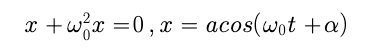where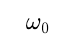is the natural oscillation frequency

2. Damped oscillation equation and its solution: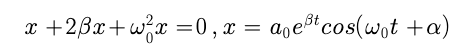where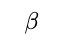is the damping coefficient,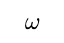is the frequency of damped oscillation;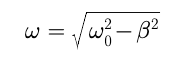3.  Logarithmic damping decrement ? and quality factor Q: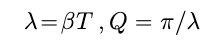where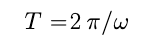4.Forced oscillation equation and its steady-state solution: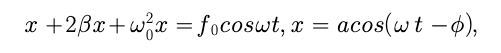Where5.Maximum shift amplitude occurs at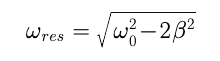Electrostatics. - Electric Field v/s distance graph & Principle of superposition

Electric Field v/s distance graph & Principle of superposition

Electrostatics - Distribution Of Charges

Electrostatics - Distribution Of Charges

FRICTION, TOPIC: Angle Of Friction And Angle Of Repose

Angle Of Friction And Angle Of Repose

FRICTION -How Much Force To Pull Up And Stop The Body

Electrostatics - Vectors Form Of Coulombs Law

Electrostatics - Vectors Form Of Coulombs Law

Laws Of Motion - Constraint Motion-

# Laws Of Motion - Constraint Motion-

Laws of motion, Topic: Third Law ( Fixed Pulley)

Laws of motion, Topic: Third Law ( Fixed Pulley)

Laws of motion, Topic: Third Law ( Movable Pulley)

Laws of motion, Topic: Third Law ( Movable Pulley)

Second Law- Calculation Of Force - Laws of motion, Topic: Second Law Of Motion

Second Law- Calculation Of Force - Laws of motion, Topic: Second Law Of Motion

First Law Of Motion (Laws Of Motion)

First Law Of Motion (Laws Of Motion)

Laws of motion - Lami's Theorem - JEE Mains

Laws of motion - Lami's Theorem - JEE Mains

basic idea of momentum and is the introduction - Laws Of Motion for IIT-JEE

The lecture gives the basic idea of momentum and is the introduction lecture for chapter Laws Of Motion for IIT-JEE.The lecture is useful for both jee-mains and jee-advance IIT-JEE 2020/2021

Law Of Gravitation. - Gravitation

Law Of Gravitation

Introduction

Newton at the age of twenty-three is said to have seen an apple falling down from tree in his orchid. This was the year 1665. He started thinking about the role of earth's attraction in the motion of moon and other heavenly bodies.  By comparing the acceleration due to gravity due to earth with the acceleration required to keep the moon in its orbit around the earth, he was able to arrive the Basic Law of Gravitation.

Newton Law Of Gravitation:

Newton's law of gravitation states that every or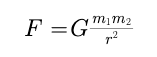Vector Form : According to Newton's law of gravitation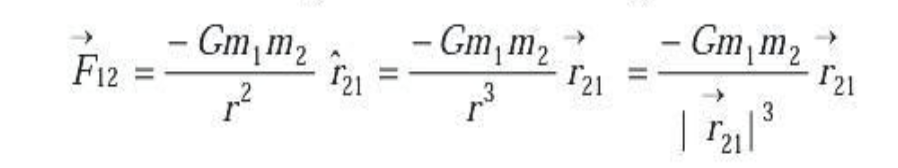body in this universe attracts every other body with a force, which is directly proportional to the product of their masses and inversely proportional to the square of the distance between their centres. The direction of the force is along the line joining the particles.  Thus the magnitude of the gravitational force F that two particles of masses m1 and m2 separated by a distance r exert on each other is given by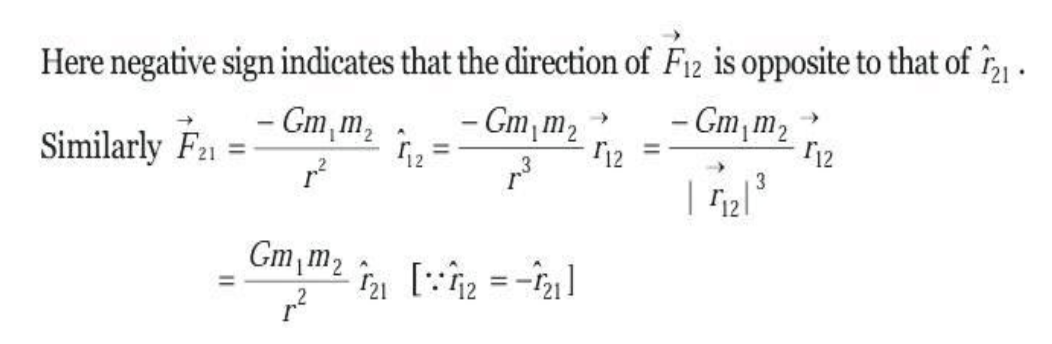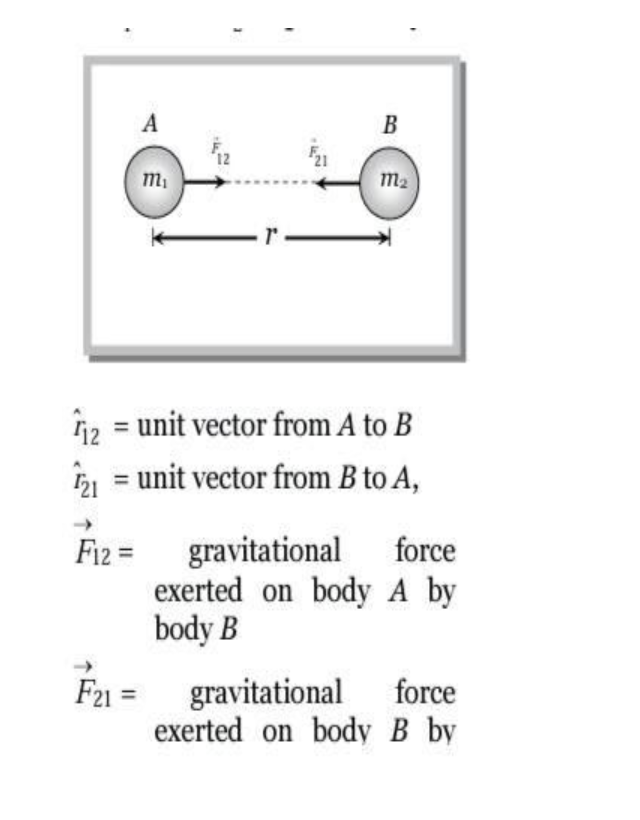universal gravitational constant is equal to the force of attraction between two bodies each of unit mass whose centres are placed unit distance apart.

Important points:

(i) The value of G in the laboratory was first determined by Cavendish using the torsional balance.

(ii) The value of G is 6.67×10–11 N–m2 kg–2 in S.I. and 6.67×10–8 dyne- cm2-g–2 in C.G.S. system.

(iii) Dimensional formula ][231TLM.

(iv) The value of G does not depend upon the nature and size of the bodies.

(v) It also does not depend upon the nature of the medium between the two bodies.

(vi) As G is very small hence gravitational forces are very small, unless one (or both) of the masses is

Properties of Gravitational Force.

(1) It is always attractive in nature while electric and magnetic force can be attractive or repulsive.

(2) It is independent of the medium between the particles while electric and magnetic force depend on the nature of the medium between the particles.

(3) It holds good over a wide range of distances. It is found true for interplanetary to inter atomic distances.

(4) It is a central force i.e. acts along the line joining the centres of two interacting bodies.

(5) It is a two-body interaction i.e. gravitational force between two particles is independent of the presence or absence of other particles; so the principle of superposition is valid i.e. force on a particle due to number of particles is the resultant of forces due to individual particles F= F1+F2+F3+…..

(7) The ratio of gravitational force to electrostatic force between two electrons is of the order of 10^-43

(8) It is a conservative force i.e. work done by it is path independent or work done in moving a particle round a closed path under the action of gravitational force is zero.

(9) It is an action reaction pair i.e. the force with which one body (say earth) attracts the second body (say moon) is equal to the force with which moon attracts the earth. This is in accordance with Newton's third law of motion.

Note :   The law of gravitation is stated for two point masses, therefore for any two arbitrary finite size bodies, as shown in the figure, It can not be applied as there is not unique value for the separation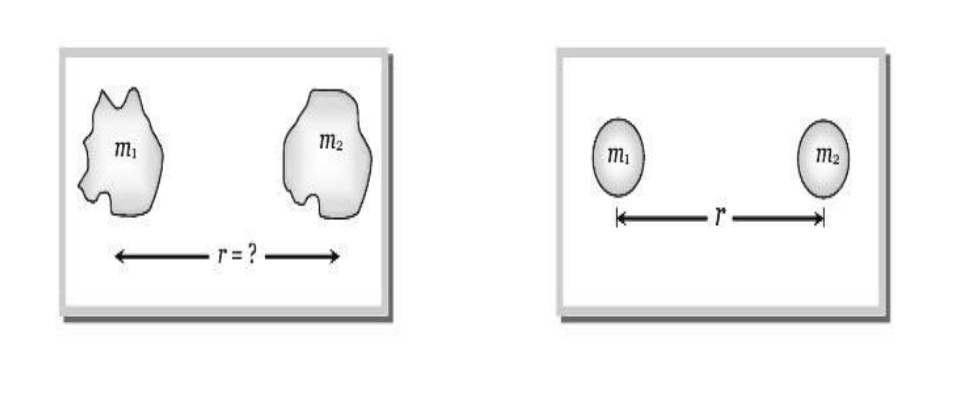But if the two bodies are uniform spheres then the separation r may be taken as the distance between their centres because a sphere of uniform mass behave as a point mass for any point lying outside it.

What is Collision. Work energy and Power

Collision

Collision is an isolated event in which a strong force acts between two or more bodies for a short time as a result of which the energy and momentum of the interacting particle change. In collision particles may or may not come in real touch e.g. in collision between two billiard balls or a ball and bat there is physical contact while in collision of alpha particle by a nucleus (i.e. Rutherford scattering experiment) there is no physical contact.

Stages of collision : There are three distinct identifiable stages in collision, namely, before, during and after. In the before and after stage the interaction forces are zero. Between these two stages, the interaction forces are very large and often the dominating forces governing the motion of bodies. The magnitude of the interacting force is often unknown,

Newton’s second law cannot be used, the law of conservation of momentum is useful in relating the initial and final velocities. (2) Momentum and energy conservation in collision :

(i) Momentum conservation : In a collision the effect of external forces such as gravity or friction are not taken into account as due to small duration of collision (t) average impulsive force responsible for collision is much larger than external force acting on the system and since this impulsive force is 'Internal' therefore the total momentum of system always remains conserved.

(ii) Energy conservation : In a collision 'total energy' is also always conserved. Here total energy includes all forms of energy such as mechanical energy, internal energy, excitation energy, radiant energy or even mass energy. These laws are the fundamental laws of physics and applicable for any type of collision but this is not true for conservation of kinetic energy.

Types of collision :

i.On the basis of conservation of kinetic energy :